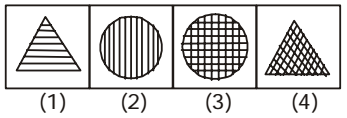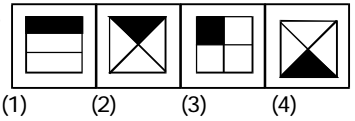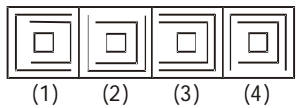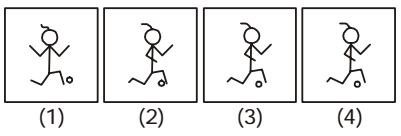## Classification

#### Classification

Direction: In each of the following questions select the one which is different from the other three responses.

1. NA1. As we can see that In all other figures there are horizontal and/or vertical lines.

##### Correct Option: D

As we can see that In all other figures there are horizontal and/or vertical lines. So , figure ( 4 ) is correct answer .

1. NA1. As we can see that In all other figures, one-fourth part of the design is shaded.

##### Correct Option: A

As we can see that In all other figures, one-fourth part of the design is shaded. In figure (1) only one-third part is shaded.

1. NA1. The open sides of the outer and middle designs face different directions except in figure (3).

##### Correct Option: C

The open sides of the outer and middle designs face different directions except in figure (3). But all others are different to figure ( 3 ) .

1. NA1. Figure (1) is different from the others.

##### Correct Option: A

Figure (1) is different from the others. But all other figures are different to figure (1) .